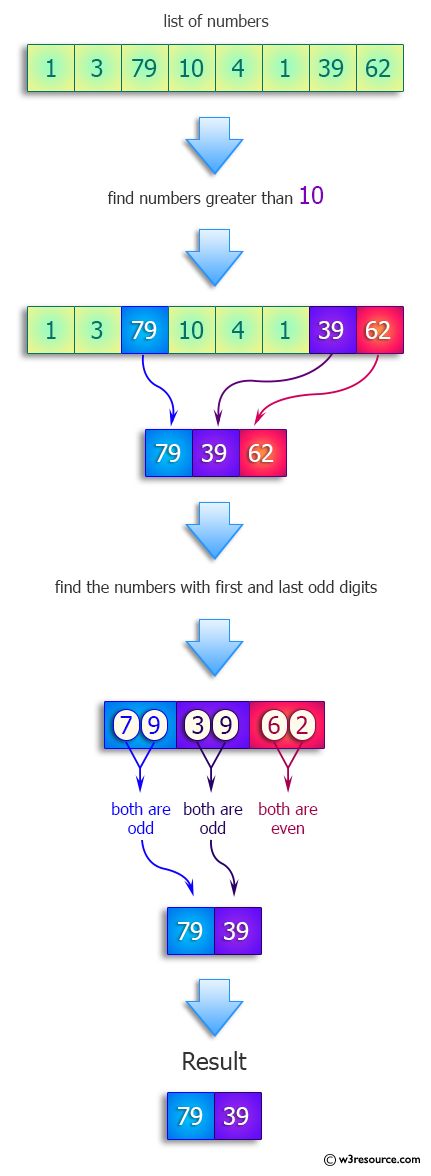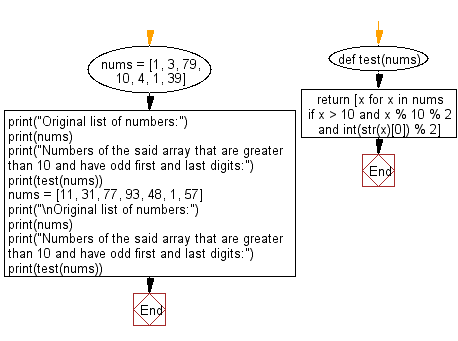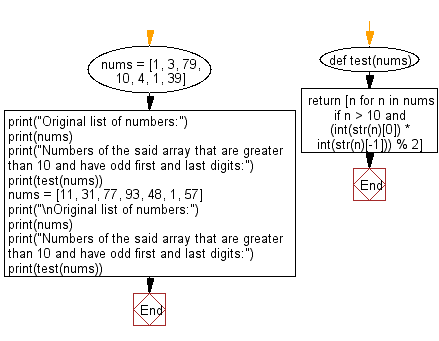﻿ Python: Find the numbers that are greater than 10 and have odd first and last digits - w3resource# Python: Find the numbers that are greater than 10 and have odd first and last digits

## Python Programming Puzzles: Exercise-55 with Solution

Write a Python program to find the numbers that are greater than 10 and have odd first and last digits.

```Input:
[1, 3, 79, 10, 4, 1, 39, 62]
Output:
[79, 39]

Input:
[11, 31, 77, 93, 48, 1, 57]
Output:
[11, 31, 77, 93, 57]
```

Pictorial Presentation:Sample Solution-1:

Python Code:

``````#License: https://bit.ly/3oLErEI

def test(nums):
return [x for x in nums if x > 10 and x % 10 % 2 and int(str(x)) % 2]

nums = [1, 3, 79, 10, 4, 1, 39]
print("Original list of numbers:")
print(nums)
print("Numbers of the said array that are greater than 10 and have odd first and last digits:")
print(test(nums))
nums = [11, 31, 77, 93, 48, 1, 57]
print("\nOriginal list of numbers:")
print(nums)
print("Numbers of the said array that are greater than 10 and have odd first and last digits:")
print(test(nums))
``````

Sample Output:

```Original list of numbers:
[1, 3, 79, 10, 4, 1, 39]
Numbers of the said array that are greater than 10 and have odd first and last digits:
[79, 39]

Original list of numbers:
[11, 31, 77, 93, 48, 1, 57]
Numbers of the said array that are greater than 10 and have odd first and last digits:
[11, 31, 77, 93, 57]
```

Flowchart:## Visualize Python code execution:

The following tool visualize what the computer is doing step-by-step as it executes the said program:

Sample Solution-2:

Python Code:

``````#License: https://bit.ly/3oLErEI

def test(nums):
return [n for n in nums if n > 10 and (int(str(n)) * int(str(n)[-1])) % 2]
nums = [1, 3, 79, 10, 4, 1, 39]
print("Original list of numbers:")
print(nums)
print("Numbers of the said array that are greater than 10 and have odd first and last digits:")
print(test(nums))
nums = [11, 31, 77, 93, 48, 1, 57]
print("\nOriginal list of numbers:")
print(nums)
print("Numbers of the said array that are greater than 10 and have odd first and last digits:")
print(test(nums))
``````

Sample Output:

```Original list of numbers:
[1, 3, 79, 10, 4, 1, 39]
Numbers of the said array that are greater than 10 and have odd first and last digits:
[79, 39]

Original list of numbers:
[11, 31, 77, 93, 48, 1, 57]
Numbers of the said array that are greater than 10 and have odd first and last digits:
[11, 31, 77, 93, 57]
```

Flowchart:## Visualize Python code execution:

The following tool visualize what the computer is doing step-by-step as it executes the said program:

Python Code Editor :

Have another way to solve this solution? Contribute your code (and comments) through Disqus.

What is the difficulty level of this exercise?

Test your Programming skills with w3resource's quiz.

﻿

## Python: Tips of the Day

Clamps num within the inclusive range specified by the boundary values x and y:

Example:

```def tips_clamp_num(num,x,y):
return max(min(num, max(x, y)), min(x, y))
print(tips_clamp_num(2, 4, 6))
print(tips_clamp_num(1, -1, -6))
```

Output:

```4
-1
```